# ML Aggarwal Solutions for Class 8 Maths Chapter 17: Visualising Solid Shapes

ML Aggarwal Solutions for Class 8 Maths Chapter 17 Visualising Solid Shapes provide students with the basic idea of understanding the solid shapes based on their views. Each question has pictorial representation with the aim of helping students analysing the problem effortlessly. For more knowledge, students can access ML Aggarwal Solutions for Class 8 Maths Chapter 17 Visualising Solid Shapes PDF, from the links which are given here.

Chapter 17 helps students in understanding the solid shapes and analysing them based on their views. The solutions are explained in simple language to help students boost their annual exam preparation, irrespective of their grasping capacity. It also provides students with confidence to solve more complex problems within the given duration.

## ML Aggarwal Solutions for Class 8 Maths Chapter 17: Visualising Solid Shapes Download PDF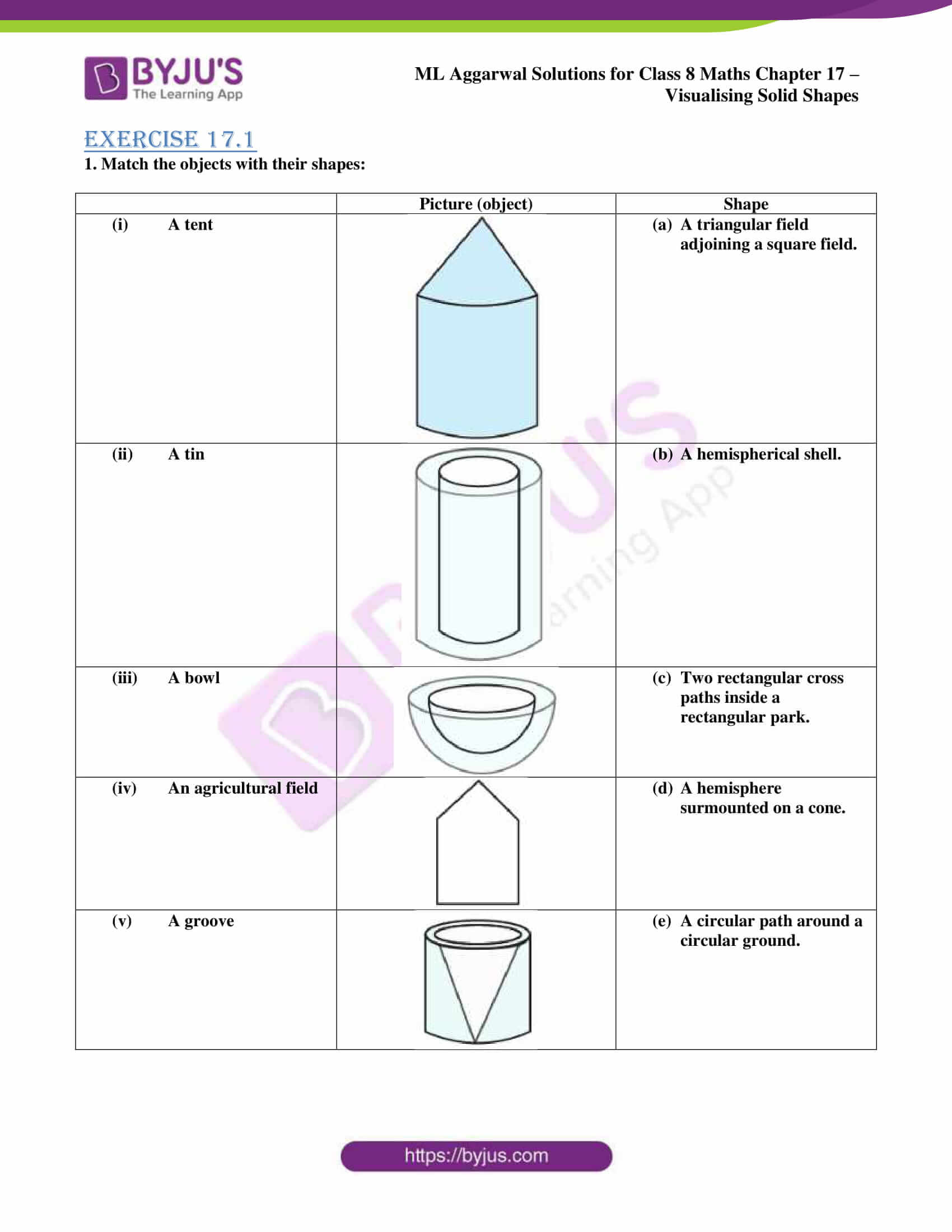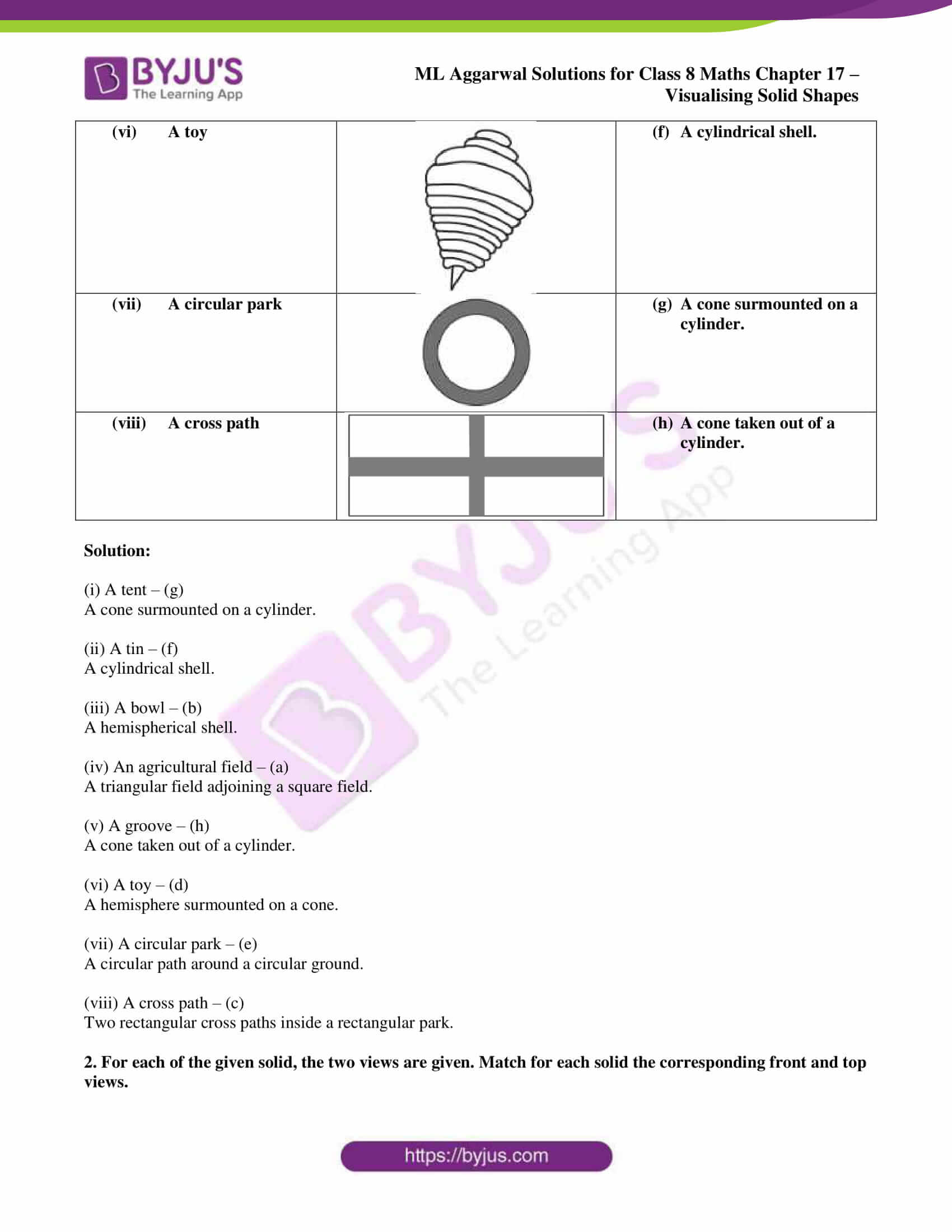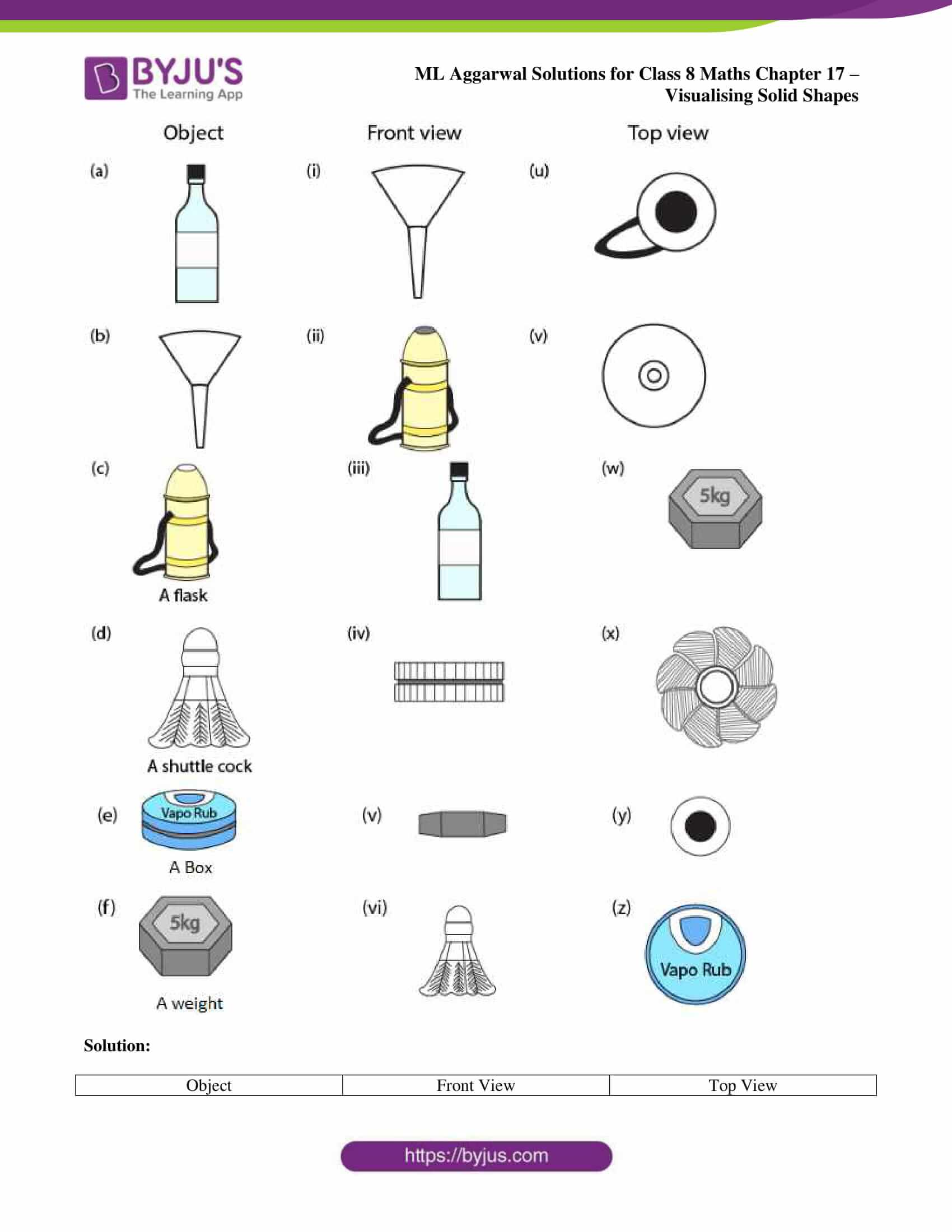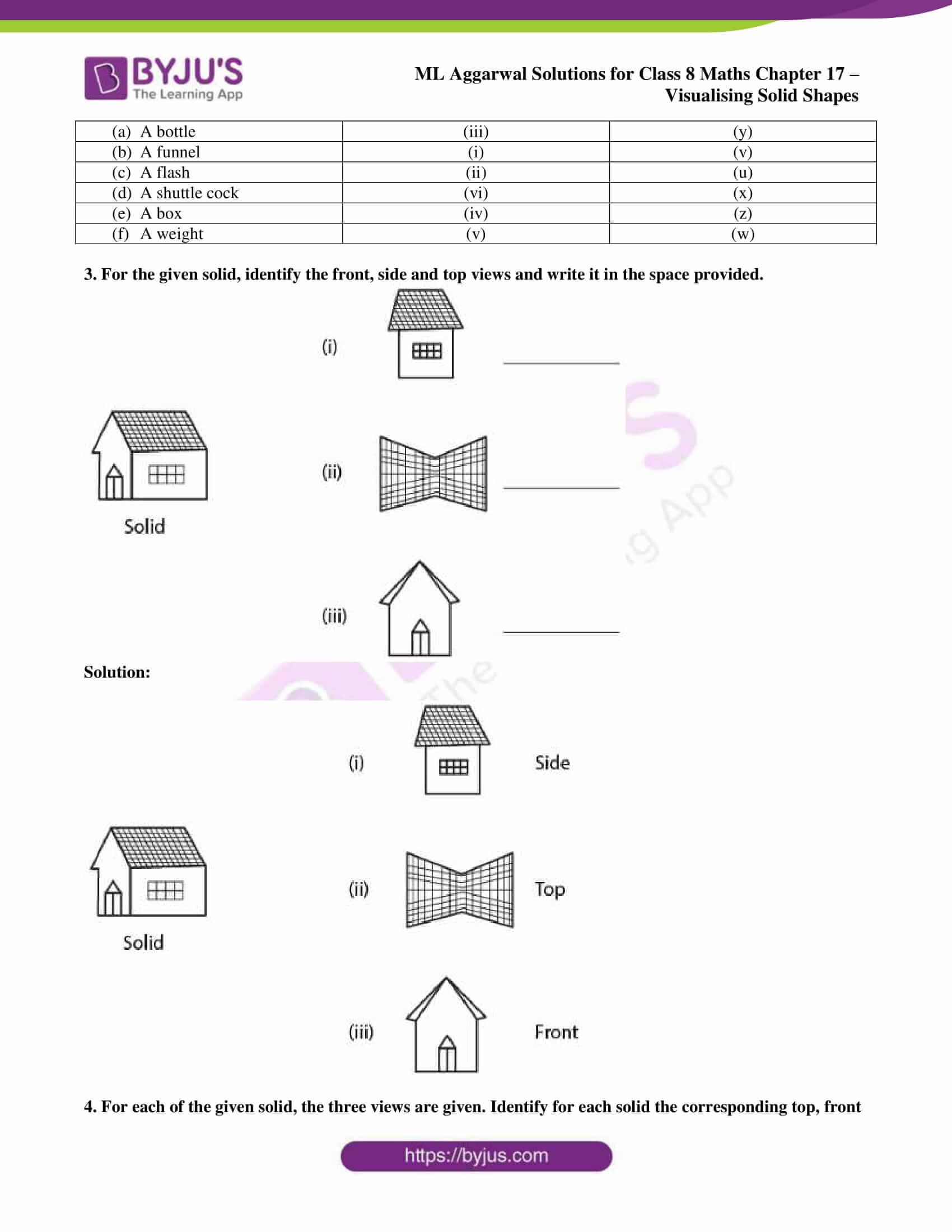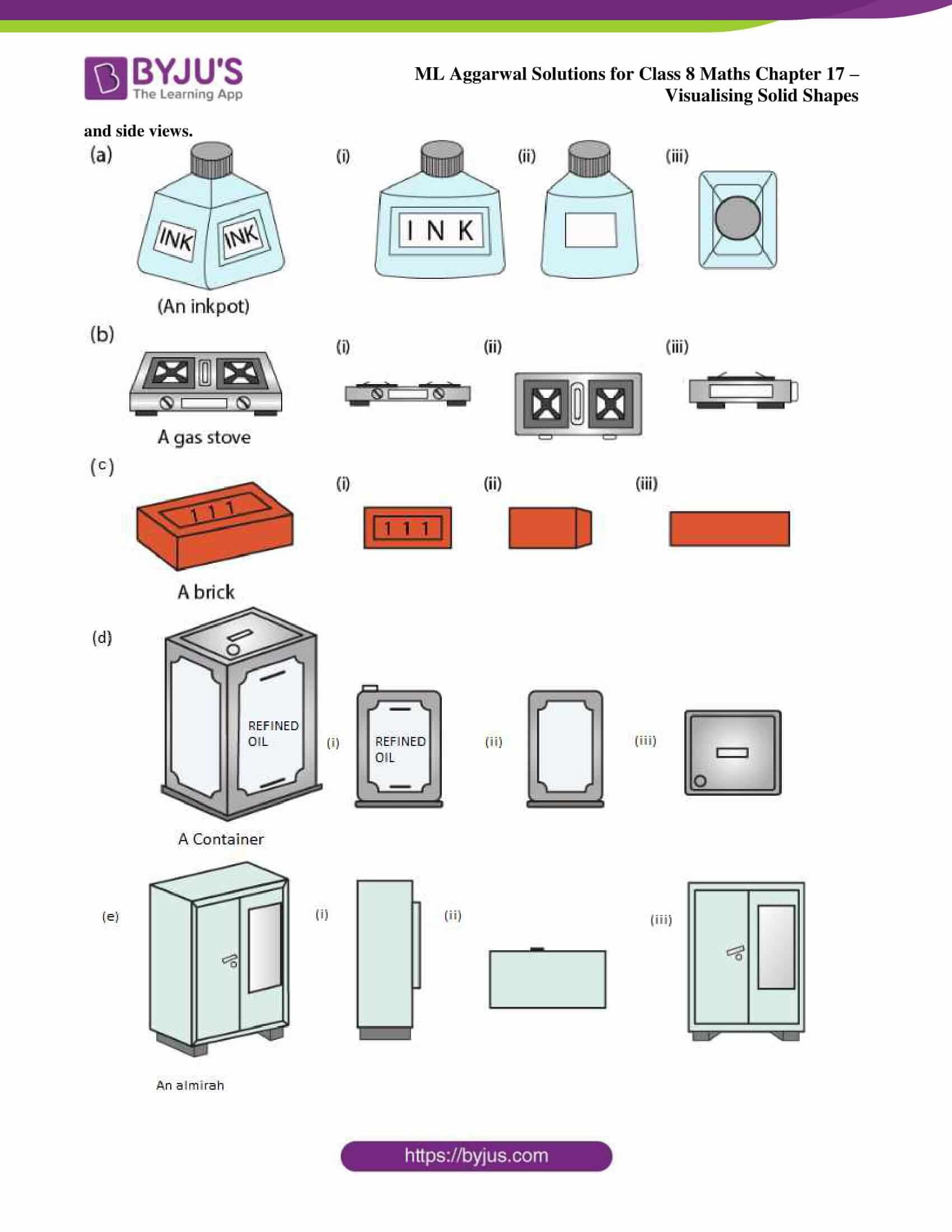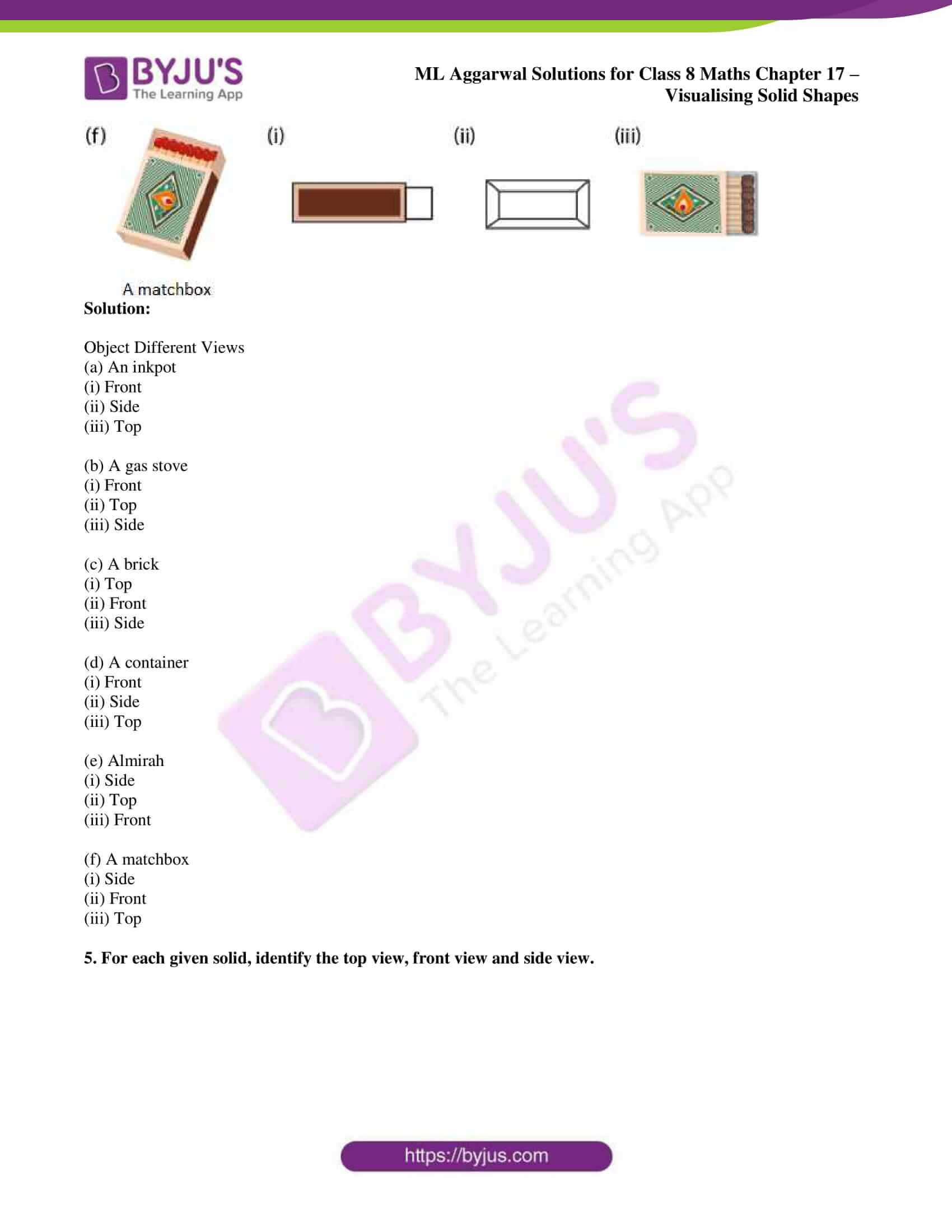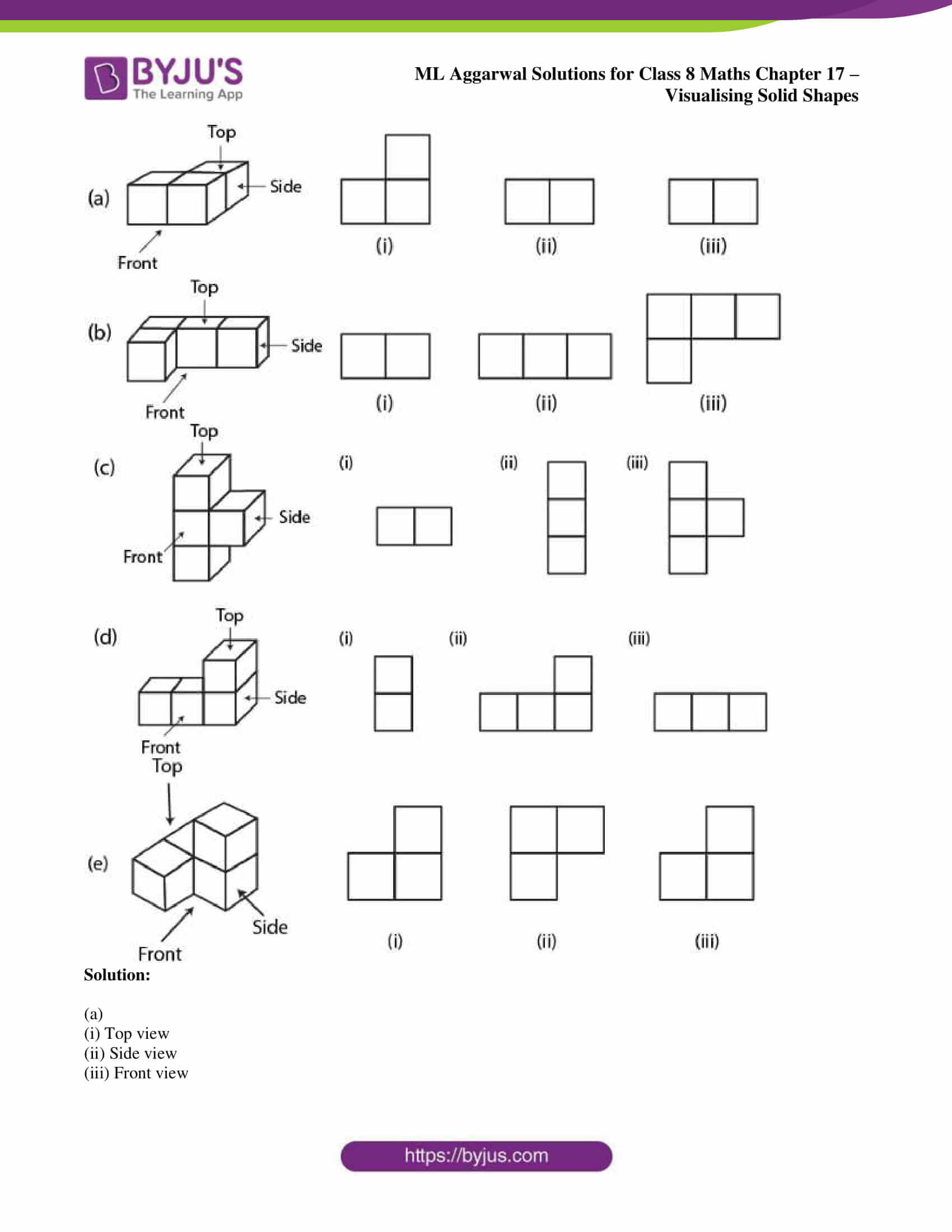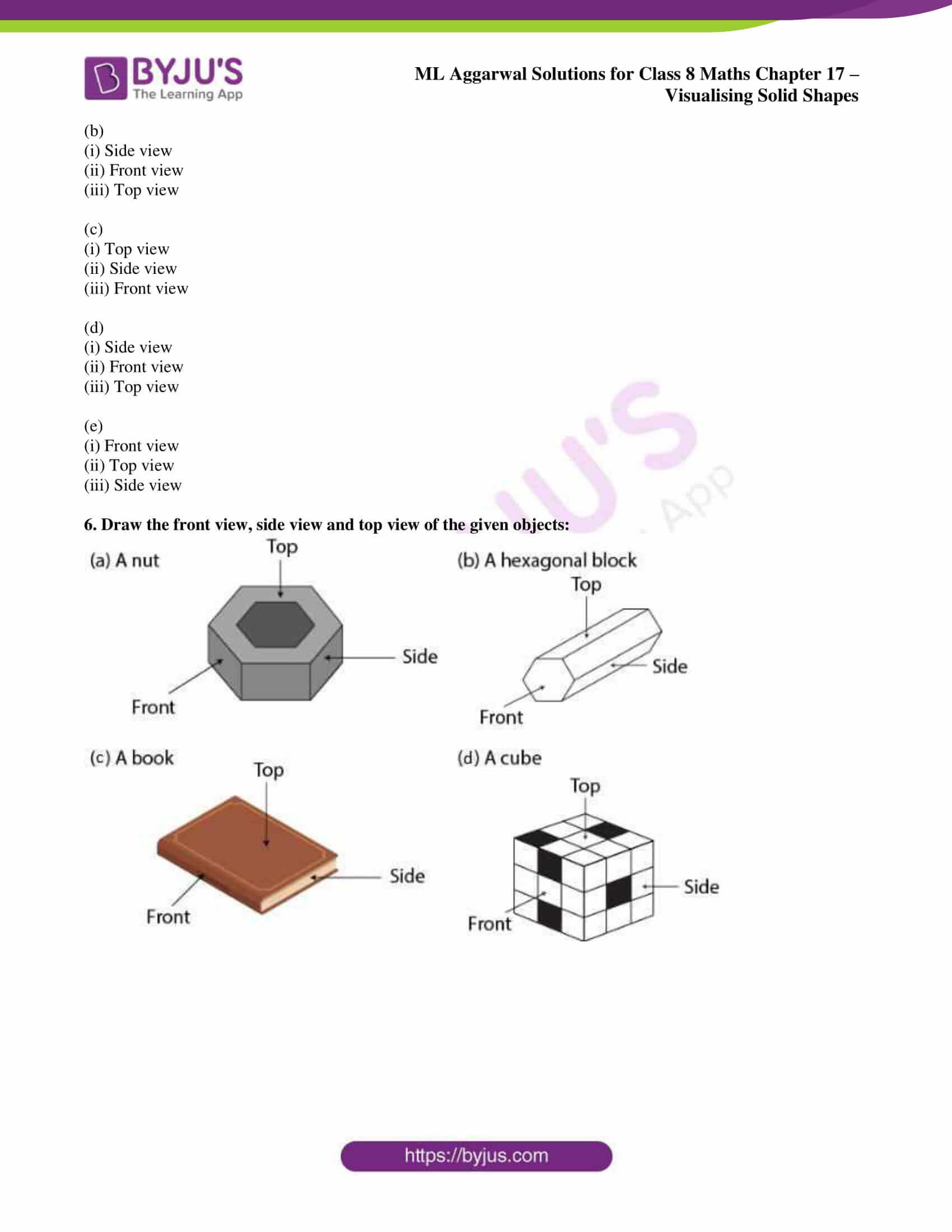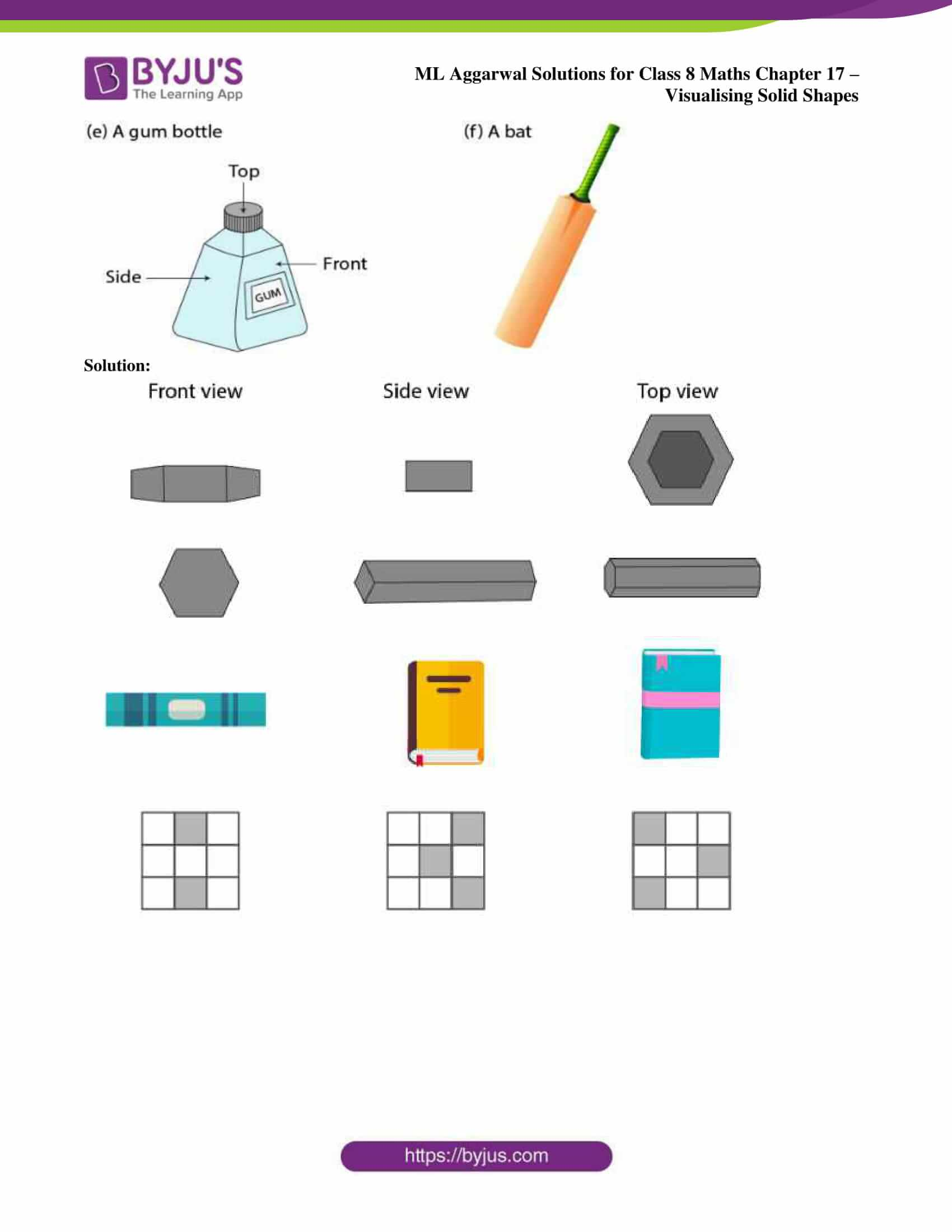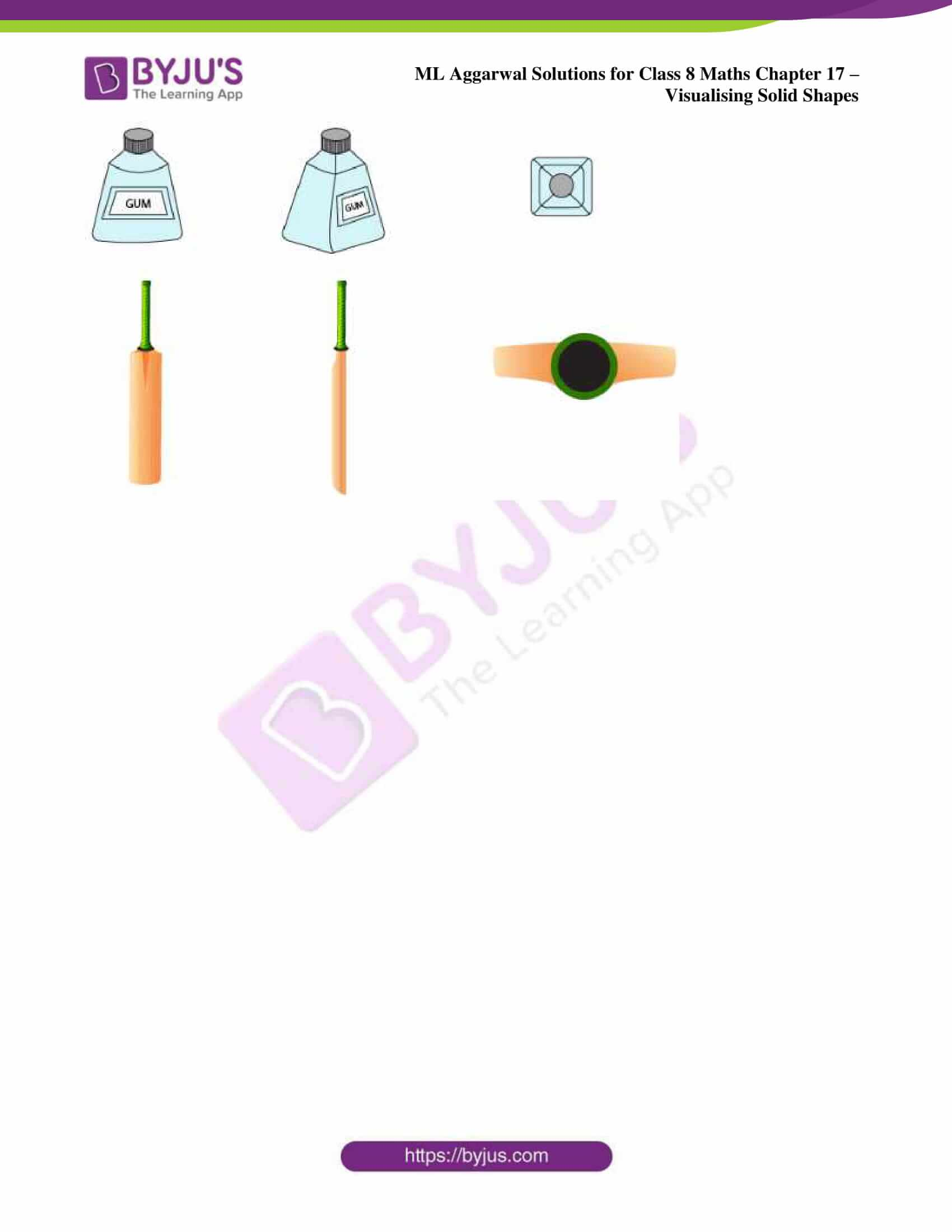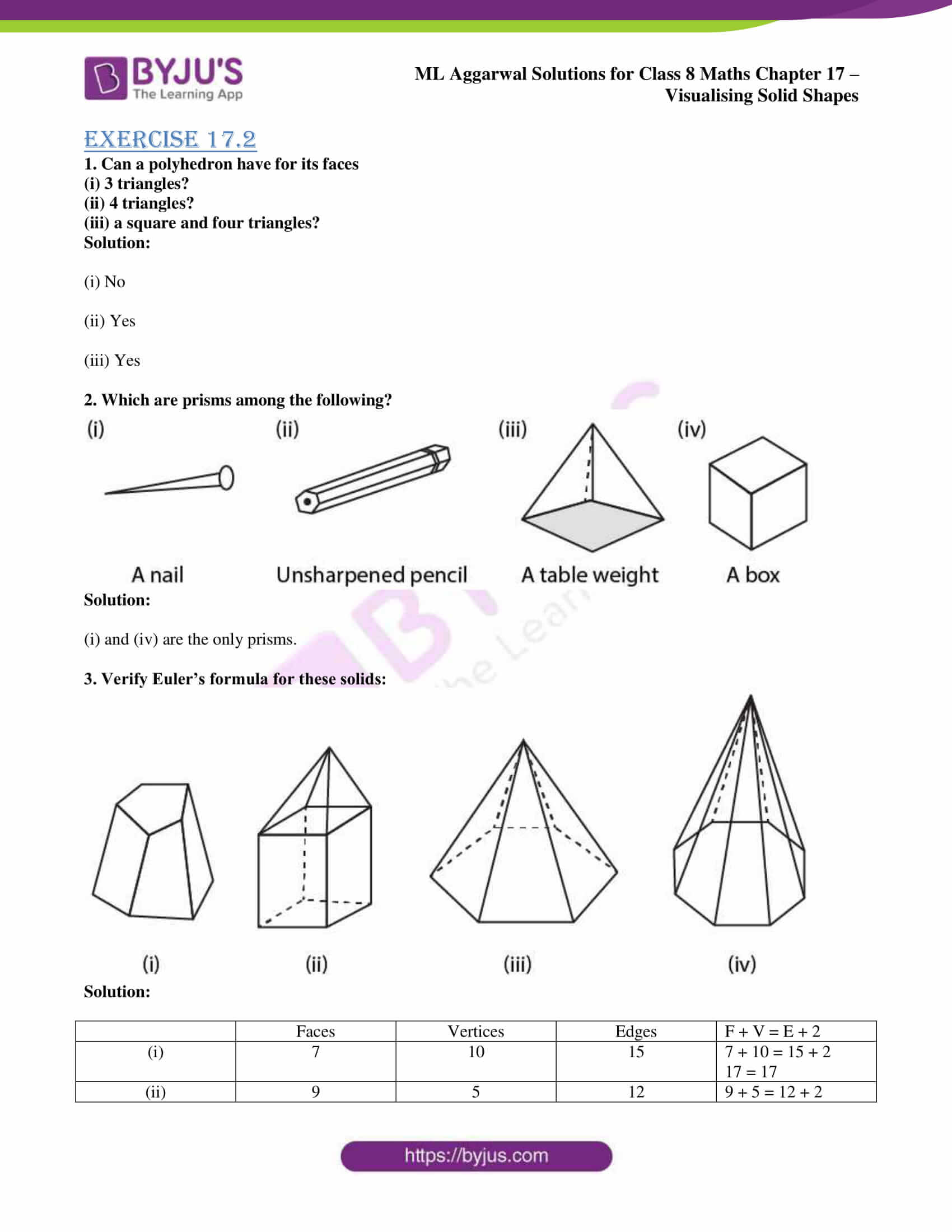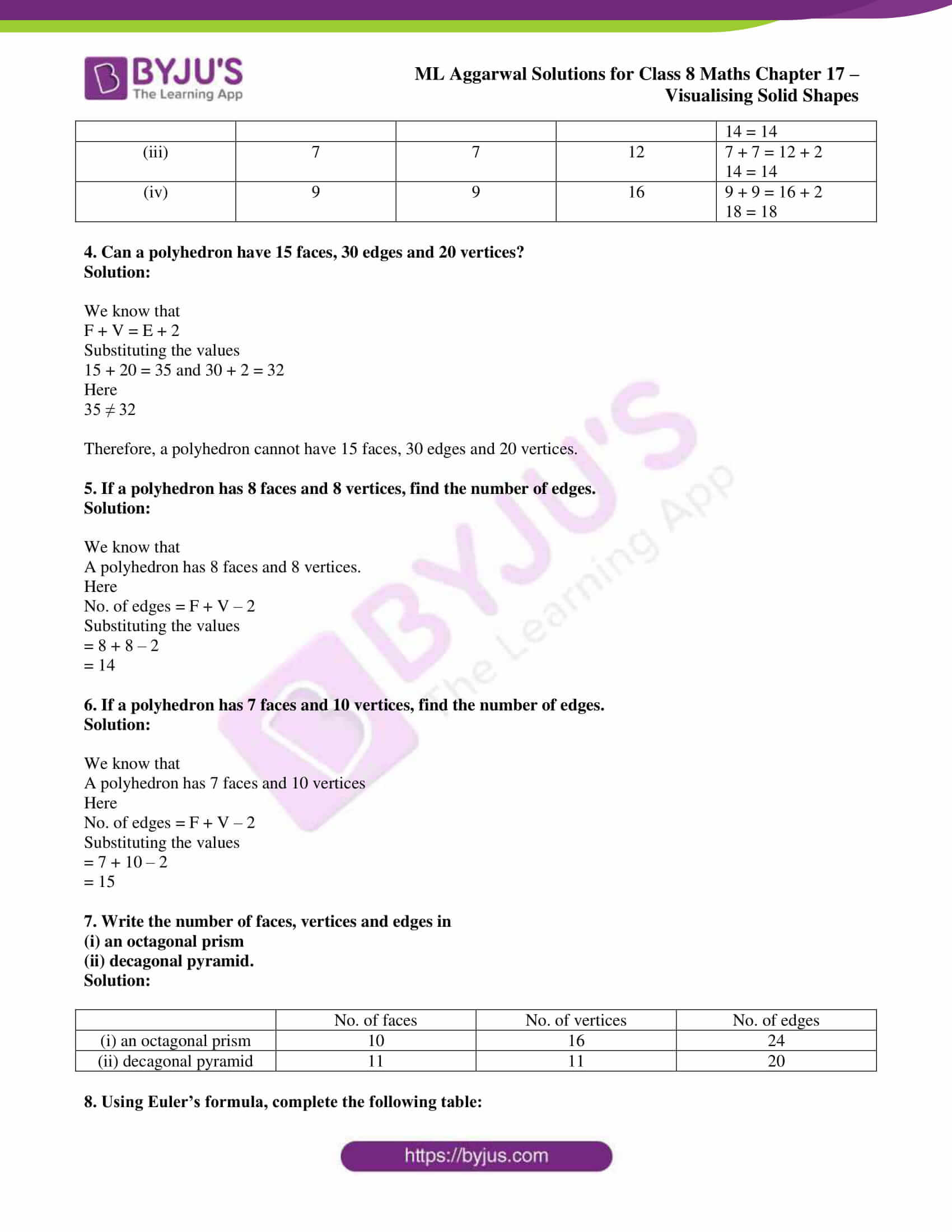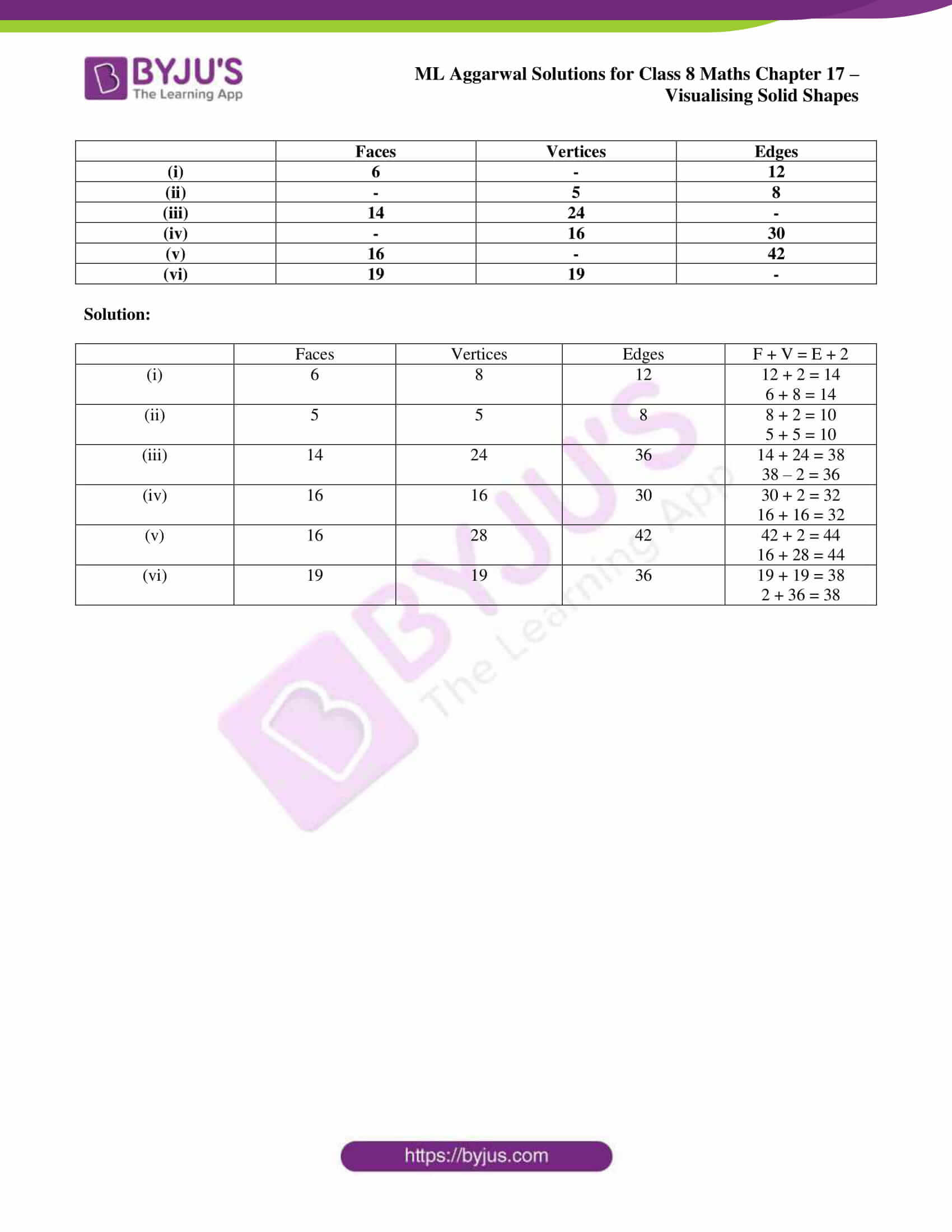## Access ML Aggarwal Solutions for Class 8 Maths Chapter 17: Visualising Solid Shapes

Exercise 17.1

1. Match the objects with their shapes:

 Picture (object) Shape A tent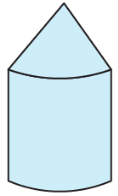A triangular field adjoining a square field. A tin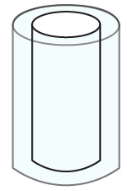A hemispherical shell. A bowl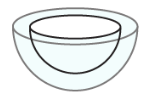Two rectangular cross paths inside a rectangular park. An agricultural field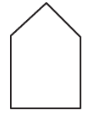A hemisphere surmounted on a cone. A groove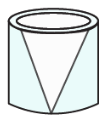A circular path around a circular ground. A toy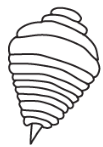A cylindrical shell. A circular park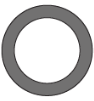A cone surmounted on a cylinder. A cross path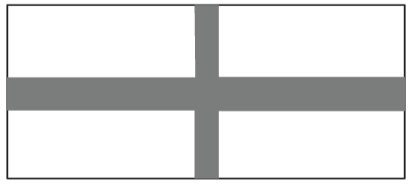A cone taken out of a cylinder.

Solution:

(i) A tent – (g)

A cone surmounted on a cylinder.

(ii) A tin – (f)

A cylindrical shell.

(iii) A bowl – (b)

A hemispherical shell.

(iv) An agricultural field – (a)

A triangular field adjoining a square field.

(v) A groove – (h)

A cone taken out of a cylinder.

(vi) A toy – (d)

A hemisphere surmounted on a cone.

(vii) A circular park – (e)

A circular path around a circular ground.

(viii) A cross path – (c)

Two rectangular cross paths inside a rectangular park.

2. For each of the given solid, the two views are given. Match for each solid the corresponding front and top views.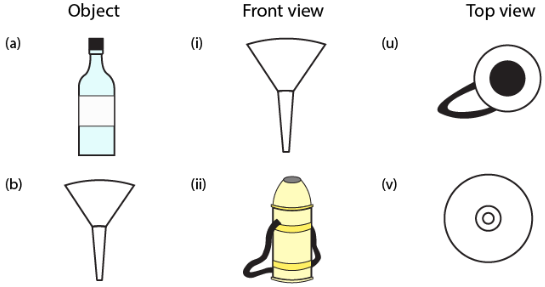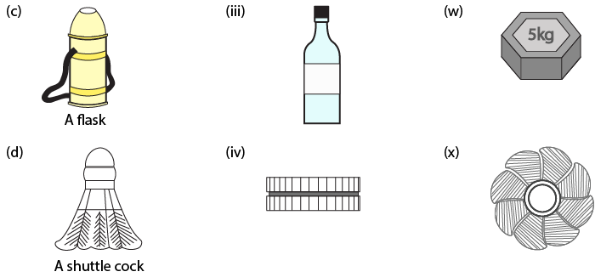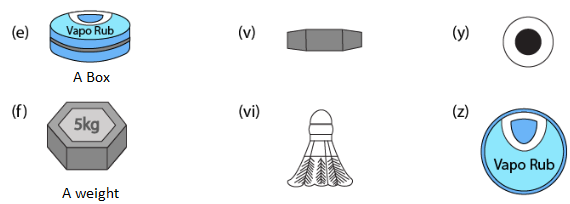Solution:

 Object Front View Top View A bottle (iii) (y) A funnel (i) (v) A flash (ii) (u) A shuttle cock (vi) (x) A box (iv) (z) A weight (v) (w)

3. For the given solid, identify the front, side and top views and write it in the space provided.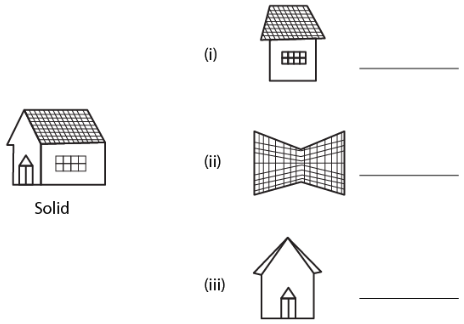Solution: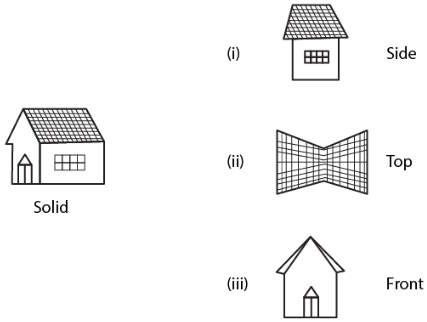4. For each of the given solid, the three views are given. Identify for each solid the corresponding top, front and side views.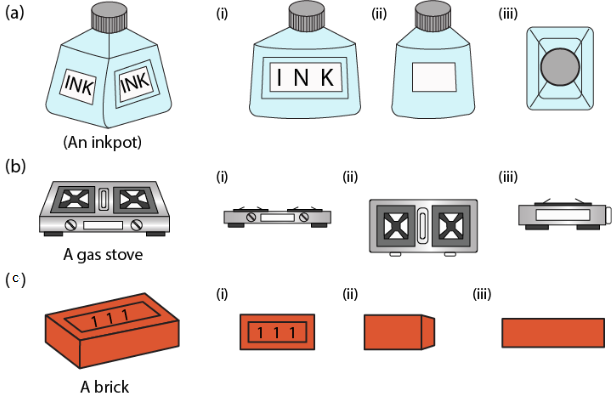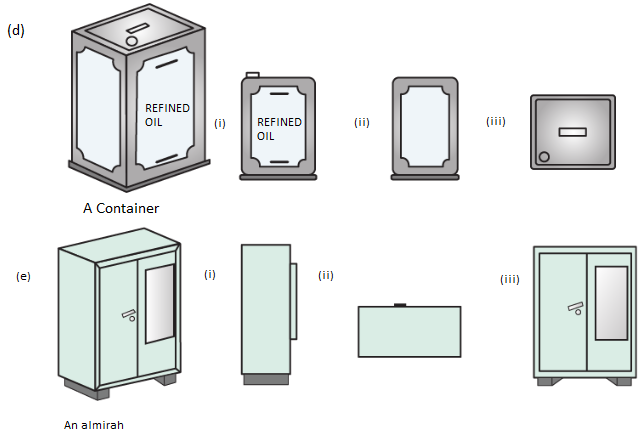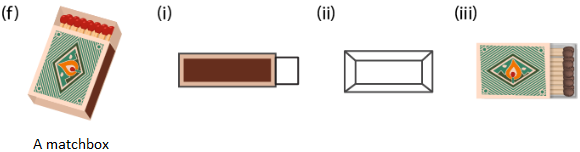Solution:

Object Different Views

(a) An inkpot

(i) Front

(ii) Side

(iii) Top

(b) A gas stove

(i) Front

(ii) Top

(iii) Side

(c) A brick

(i) Top

(ii) Front

(iii) Side

(d) A container

(i) Front

(ii) Side

(iii) Top

(e) Almirah

(i) Side

(ii) Top

(iii) Front

(f) A matchbox

(i) Side

(ii) Front

(iii) Top

5. For each given solid, identify the top view, front view and side view.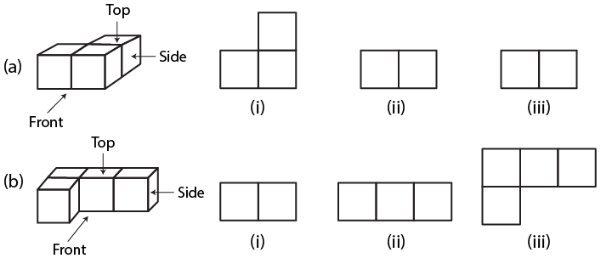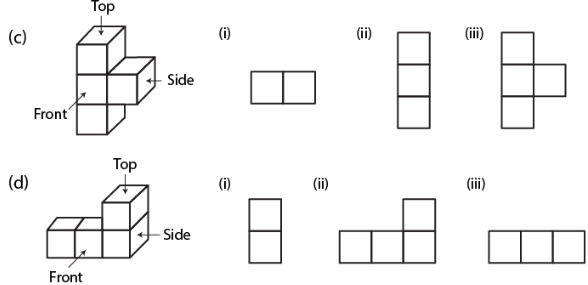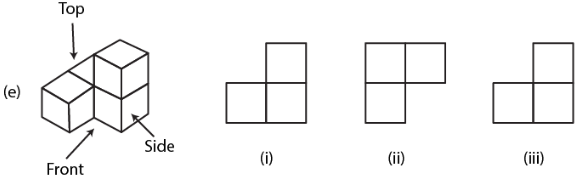Solution:

(a)

(i) Top view

(ii) Side view

(iii) Front view

(b)

(i) Side view

(ii) Front view

(iii) Top view

(c)

(i) Top view

(ii) Side view

(iii) Front view

(d)

(i) Side view

(ii) Front view

(iii) Top view

(e)

(i) Front view

(ii) Top view

(iii) Side view

6. Draw the front view, side view and top view of the given objects: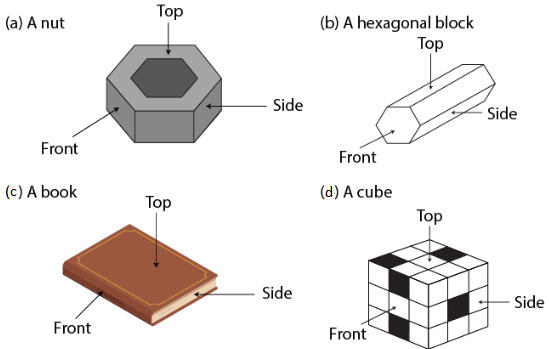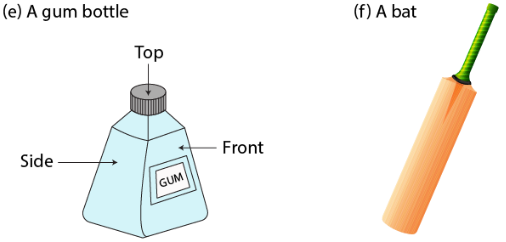Solution: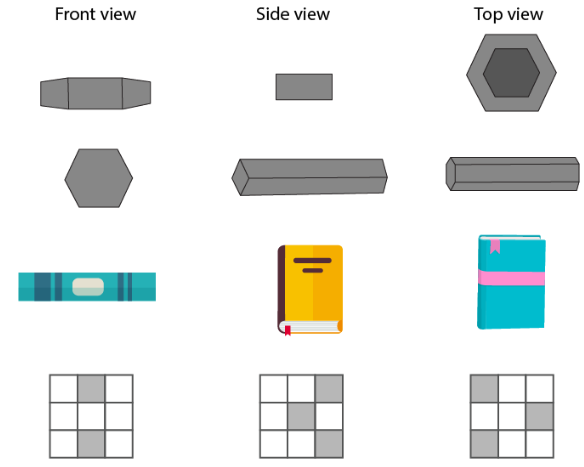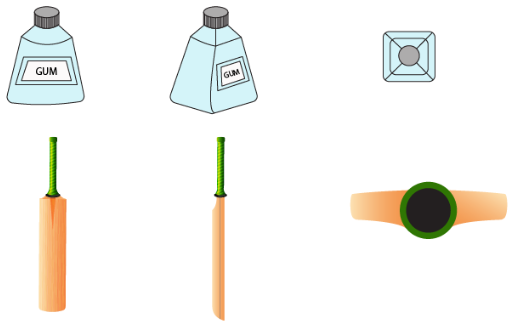Exercise 17.2

1. Can a polyhedron have for its faces

(i) 3 triangles?

(ii) 4 triangles?

(iii) a square and four triangles?

Solution:

(i) No

(ii) Yes

(iii) Yes

2. Which are prisms among the following?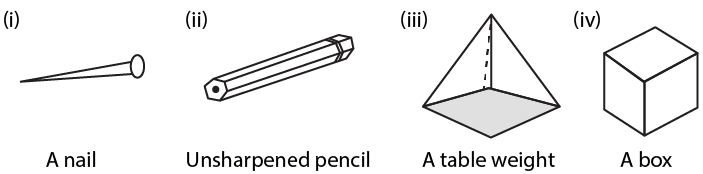Solution:

(i) and (iv) are the only prisms.

3. Verify Euler’s formula for these solids: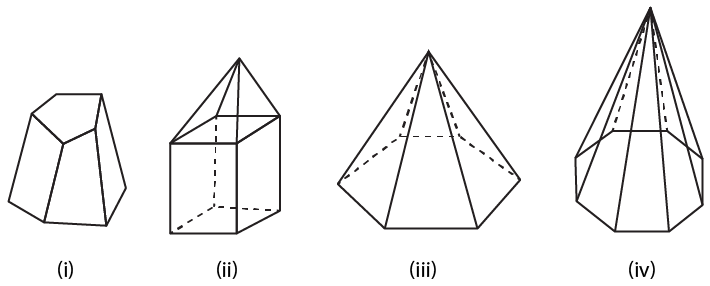Solution:

 Faces Vertices Edges F + V = E + 2 (i) 7 10 15 7 + 10 = 15 + 2 17 = 17 (ii) 9 5 12 9 + 5 = 12 + 2 14 = 14 (iii) 7 7 12 7 + 7 = 12 + 2 14 = 14 (iv) 9 9 16 9 + 9 = 16 + 2 18 = 18

4. Can a polyhedron have 15 faces, 30 edges and 20 vertices?

Solution:

We know that

F + V = E + 2

Substituting the values

15 + 20 = 35 and 30 + 2 = 32

Here

35 ≠ 32

Therefore, a polyhedron cannot have 15 faces, 30 edges and 20 vertices.

5. If a polyhedron has 8 faces and 8 vertices, find the number of edges.

Solution:

We know that

A polyhedron has 8 faces and 8 vertices.

Here

No. of edges = F + V – 2

Substituting the values

= 8 + 8 – 2

= 14

6. If a polyhedron has 7 faces and 10 vertices, find the number of edges.

Solution:

We know that

A polyhedron has 7 faces and 10 vertices

Here

No. of edges = F + V – 2

Substituting the values

= 7 + 10 – 2

= 15

7. Write the number of faces, vertices and edges in

(i) an octagonal prism

(ii) decagonal pyramid.

Solution:

 No. of faces No. of vertices No. of edges (i) an octagonal prism 10 16 24 (ii) decagonal pyramid 11 11 20

8. Using Euler’s formula, complete the following table:

 Faces Vertices Edges (i) 6 – 12 (ii) – 5 8 (iii) 14 24 – (iv) – 16 30 (v) 16 – 42 (vi) 19 19 –

Solution:

 Faces Vertices Edges F + V = E + 2 (i) 6 8 12 12 + 2 = 14 6 + 8 = 14 (ii) 5 5 8 8 + 2 = 10 5 + 5 = 10 (iii) 14 24 36 14 + 24 = 38 38 – 2 = 36 (iv) 16 16 30 30 + 2 = 32 16 + 16 = 32 (v) 16 28 42 42 + 2 = 44 16 + 28 = 44 (vi) 19 19 36 19 + 19 = 38 2 + 36 = 38